# Algebraic Equations SOLVING MULTISTEP EQUATIONS March 1 2006

• Slides: 12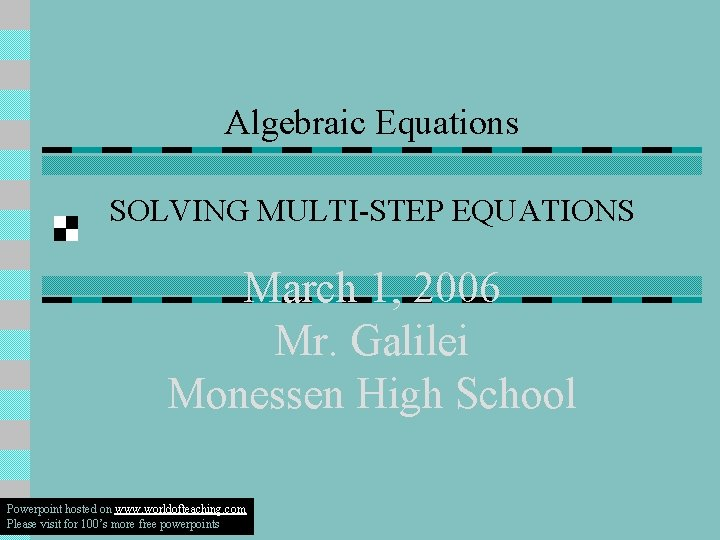Algebraic Equations SOLVING MULTI-STEP EQUATIONS March 1, 2006 Mr. Galilei Monessen High School Powerpoint hosted on www. worldofteaching. com Please visit for 100’s more free powerpoints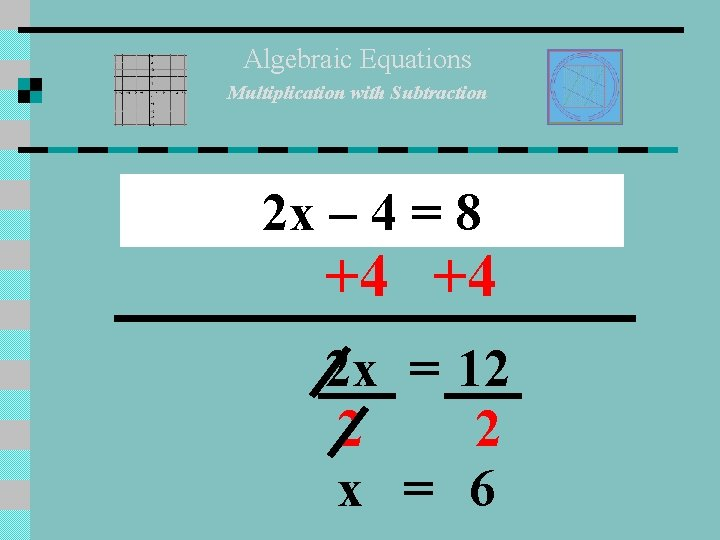Algebraic Equations Multiplication with Subtraction 2 x – 4 = 8 +4 +4 2 x = 12 2 2 x = 6Algebraic Equations Multiplication with Addition 5 x + 10 = 80 -10 5 x = 70 5 5 x = 14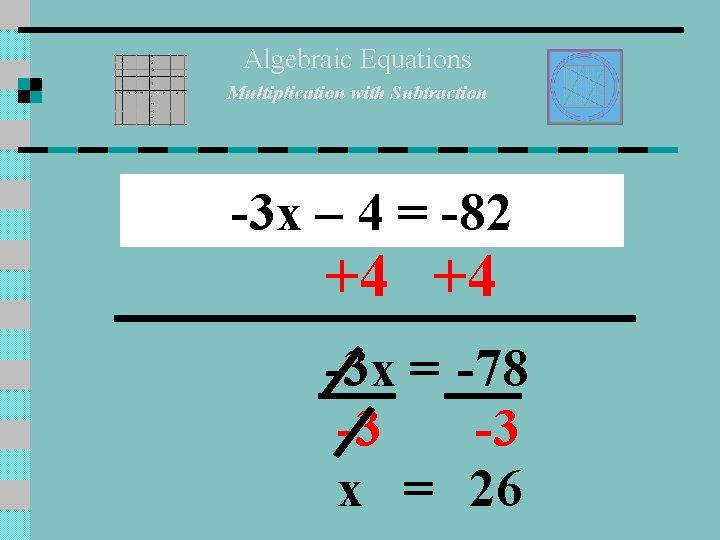Algebraic Equations Multiplication with Subtraction -3 x – 4 = -82 +4 +4 -3 x = -78 -3 -3 x = 26Algebraic Equations Multiplication with Addition 2/3 x + 2 = 8 -2 -2 (HINT) Reciprocal 3 2 2 3 x = 6 3 2 x = 18/2 = 9Algebraic Equations Division with Addition x/5 + 2 = 8 -2 -2 x (5) 5 = 6 (5) x = 30Algebraic Equations C. L. T. (4 x +6 x )+ 20 = 80 10 x + 20 = 80 -20 10 x = 60 10 10 x=6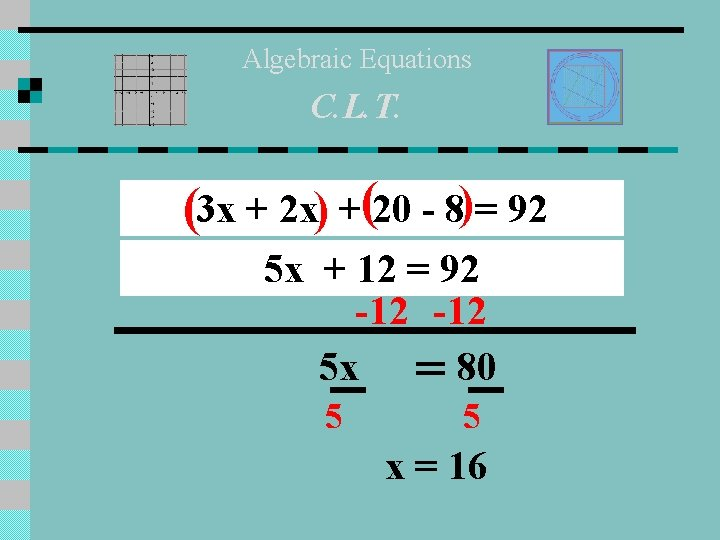Algebraic Equations C. L. T. (3 x + 2 x) +(20 - 8)= 92 5 x + 12 = 92 -12 5 x = 80 5 5 x = 16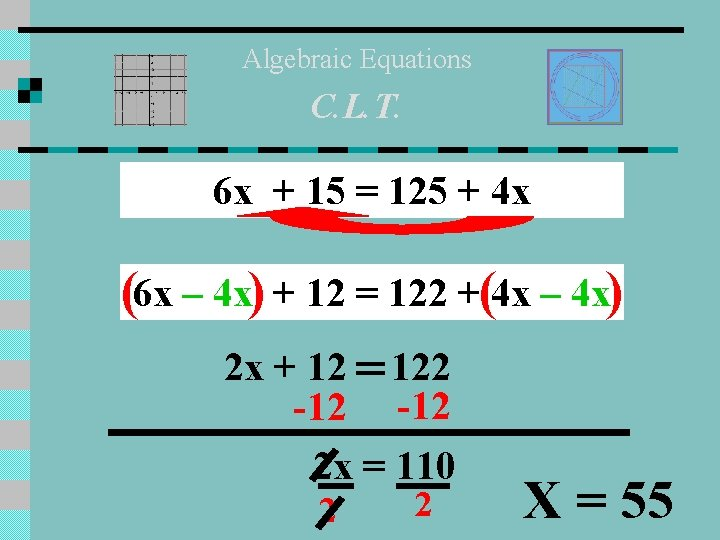Algebraic Equations C. L. T. 6 x + 15 = 125 + 4 x (6 x – 4 x) + 12 = 122 +(4 x – 4 x) 2 x + 12 = 122 -12 2 x = 110 2 2 X = 55Algebraic Equations Distributive Property 2(5 x – 6) – 3 x = 4(x + 12) – 9 x 10 x – 12 - 3 x = 4 x + 48 – 9 x (10 x – 3 x)-12 =(4 x – 9 x)+ 48 7 x - 12 = -5 x + 48 (7 x + 5 x)- 12 = 48 12 x - 12 = 48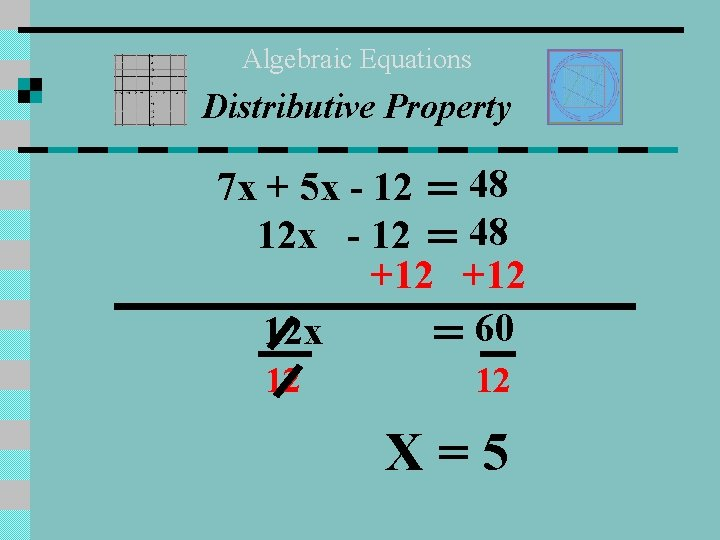Algebraic Equations Distributive Property 7 x + 5 x - 12 = 48 12 x - 12 = 48 +12 12 x = 60 12 12 X=5THE END n Homework: Study Review Sheet Use Worksheets & Notes to review and practice for the test.